Courses

# Unit - II Time Series CA Foundation Notes | EduRev

## Business Mathematics and Logical Reasoning & Statistics

Created by: Sushil Kumar

## CA Foundation : Unit - II Time Series CA Foundation Notes | EduRev

The document Unit - II Time Series CA Foundation Notes | EduRev is a part of the CA Foundation Course Business Mathematics and Logical Reasoning & Statistics.
All you need of CA Foundation at this link: CA Foundation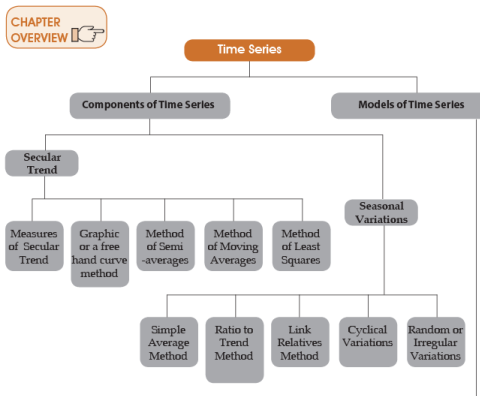19.2.1 INTRODUCTION:- We came across a data which is collected on a variable/s (rainfall, production of industrial product, production of rice, sugar cane, import/export of a country, population, etc.) at different time epochs (hours, days, months, years etc.), such a data is called time series data. Time series is statistical data that are arranged and presented in a chronological order i.e., over a period of time.
Most of the time series relating to Economic, Business and Commerce might show an upward tendency in case of population, production & sales of products, incomes, prices; or downward tendency might be noticed in time series relating to share prices, death, birth rate etc. due to global melt down, or improvement in medical facilities etc.
Definition: According to Spiegel, “A time series is a set of observations taken at specific ed times, usually at equal intervals.” According to Ya-Lun-Chou, “A time series may be defined as a collection of reading belonging to different time period of same economic variable or composite of variables.”
Components of Time Series: There are various forces that affect the values of a phenomenon in a time series; these may be broadly divided into the following four categories, commonly known as the components of a time series.
(1) Long term movement or Secular Trend
(2) Seasonal variations
(3) Cyclical variations
(4) Random or irregular variations
In traditional or classical time series analysis, it is ordinarily assumed that there are:
1. Secular Trend or Simple trend:-

Secular trend is the long: Term tendency of the time series to move in an upward or down ward direction. It indicates how on the whole, it has behaved over the entire period under reference. These are result of long-term forces that gradually operate on the time series variable. A general tendency of a variable to increase, decrease or remain constant in long term (though in a small time interval it may increase or decrease) is called trend of a variable. E.g. Population of a country has increasing trend over a years. Due to modern technology, agricultural and industrial production is increasing. Due to modern technology health facilities, death rate is decreasing and life expectancy is increasing. Secular trend is be long-term tendency of the time series to move on upward or downward direction. It indicates how on the whole behaved over the entire period under reference. These are result of long term forces that gradually operate on the time series variable. A few examples of theses long term forces which make a time series to move in any direction over long period of the time are long term changes per capita income, technological improvements of growth of population, Changes in Social norms etc.
Most of the time series relating to Economic, Business and Commerce might show an upward tendency in case of population, production & sales of products, incomes, prices; or downward tendency might be noticed in time series relating to share prices, death, birth rate etc. due to global melt down, or improvement in medical facilities etc. All these indicate trend.
2. Seasonal variations:- Over a span of one year, seasonal variation takes place due to the rhythmic forces which operate in a regular and periodic manner. These forces have the same or almost similar pattern year after year.
It is common knowledge that the value of many variables depends in part on the time of year. For Example, Seasonal variations could be seen and calculated if the data are recorded quarterly, monthly, weekly, daily or hourly basis. So if in a time series data only annual figures are given, there will be no seasonal variations.
The seasonal variations may be due to various seasons or weather conditions for example sale of cold drink would go up in summers & go down in winters. These variations may be also due to man-made conventions & due to habits, customs or traditions. For example, sales might go up during Diwali & Christmas or sales of restaurants & eateries might go down during Navratri’s.
The method of seasonal variations are
(i) Simple Average Method
(ii) Ratio to Trend Method
(iii) Ratio to Moving Average Method
3. Cyclical variations:- Cyclical variations, which are also generally termed as business cycles, are the periodic movements.  These variations in a time series are due to ups & downs recurring after a period from Season to Season. Though they are more or less regular, they may not be uniformly periodic. These are oscillatory movements which are present in any business activity and is termed as business cycle. It has got four phases consisting of prosperity (boom), recession, depression and recovery. All these phases together may last from 7 to 9 years may be less or more.
4. Random or irregular variations:- These are irregular variations which occur on account of random external events. These variations either go very deep downward or too high upward to attain peaks abruptly. These fluctuations are a result of unforeseen and unpredictably forces which operate in absolutely random or erratic manner. They do not have any definite pattern and it cannot be predicted in advance. These variations are due to floods, wars, famines, earthquakes, strikes, lockouts, epidemics etc.

19.2.2 MODELS OF TIME SERIES:- The following are the two models which are generally used for decomposition of time series into its four components. The objective is to estimate and separate the four types of variations and to bring out the relative impact of each on the overall behaviour of the time series.
(2) Multiplicative model
Additive Model: In additive model it is assumed that the four components are independent of one another i.e. the pattern of occurrence and magnitude of movements in any particular component does not affect and are not affected by the other component. Under this assumption the four components are arithmetically additive ie. magnitude of time series is the sum of the separate inß uences of its four components i.e. Yt= T + C + S + I
Where,
Yt = Time series
T = Trend variation
C = Cyclical variation
S = Seasonal variation
I = Random or irregular variation
Multiplicative Model: In this model it is assumed that the forces that give rise to four types of variations are interdependent, so that overall pattern of variations in the time series is a combined result of the interaction of all the forces operating on the time series. Accordingly, time series are the product of its four components i.e.
Yt = T x C x S x I
As regards to the choice between the two models, it is generally the multiplication model which is used more frequently. As the forces responsible for one type of variation are also responsible for other type of variations, hence it is multiplication model which is more suited in most business and economic time series data for the purpose of decomposition.

Example 19.2.1: Under the additive model, a monthly sale of ₹21,110 explained as follows:
The trend might be ₹20,000, the seasonal factor: ₹1,500 (The month question is a good one for sales, expected to be ₹1,500 over the trend), the cyclical factor: ₹800 (A general Business slump is experienced, expected to depress sales by ₹800 (per month); and Residual Factor: ₹410 (Due to unpredictable random ß uctuations).
The model gives:
Y = T + C + S + R
21,110 = 2,000 + 1,500 + (-800) + 410
The multiplication model might explain the same sale figure in similar way.
Trend = ₹20,000, Seasonal Factor: ₹1.15 (a good month for sales, expected to be 15 per cent above the trend)
Cyclical Factor: 0.90 (a business slump, expected to cause a 10 per cent reduction in sales) and
Residual Factor: 1.02 (Random ß uctuations of + 2 Factor)
Y = T × S × C × R
21114 = 20,000 × 1.15 × 0.90 × 1.02

19.2.3 MEASUREMENT OF SECULAR TREND:- The following are the methods most commonly used for studying & measuring the trend component in a time series -
(1) Graphic or a Freehand Curve Method
(2) Method of Semi Averages
(3) Method of Moving Averages
(4) Method of Least Squares
Graphic or Freehand Curve Method:-
The data of a given time series is plotted on a graph and all the points are joined together with a straight line. This curve would be irregular as it includes short run oscillation. These irregularities are smoothened out by drawing a freehand curve or line along with the curve previously drawn.
This curve would eliminate the short run oscillations and would show the long period general tendency of the data. While drawing this curve it should be kept in mind that the curve should be smooth and the number of points above the trend curve should be more or less equal to the number of points below it.
Merits:- (1) It is very simple and easy to understand
(2) It does not require any mathematical calculations
(1) This is a subjective concept. Hence different persons may draw freehand lines at different positions and with different slopes.
(2)  If the length of period for which the curve is drawn is very small, it might give totally erroneous results.
Example 19.2.2:- The following are figures of a Sale for the last nine years. Determine the trend by line by the freehand method.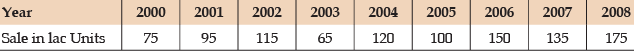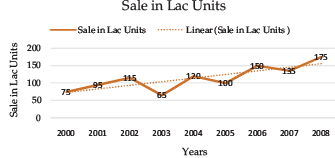The trend line drawn by the freehand method can be extended to predicted values. However, since the freehand curve fitting is too subjective, the method should not be used as basis for predictions.
Methods of Semi averages: Under this method the whole time series data is classiÞ ed into two equal parts and the averages for each half are calculated. If the data is for even number of years, it is easily divided into two. If the data is for odd number of years, then the middle year of the time series is left and the two halves are constituted with the period on each side of the middle year.
The arithmetic mean for a half is taken to be representative of the value corresponding to the midpoint of the time interval of that half. Thus we get two points. These two points are plotted on a graph and then are joined by straight line which is our required trend line.
Example 19.2.3: Fit a trend line to the following data by the method of Semi-averages.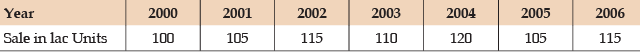Solution: Since the data consist of seven Years, the middle year shall be left out and an average of the first three years and last three shall be obtained. The average of first three years is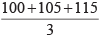or 320/3 or 106.67 and the average of last three years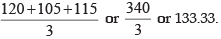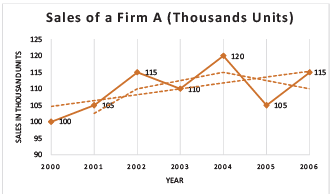Moving average method: A moving average is an average (Arithmetic mean) of fixed number of items (known as periods) which moves through a series by dropping the first item of the previously averaged group and adding the next item in each successive average. The value so computed is considered the trend value for the unit of time falling at the centre of the period used in the calculation of the average.
In case the period is odd: If the period of moving average is odd for instance for computing 3 yearly moving average, the value of 1st, 2nd and 3rd years are added up and arithmetic mean is found out and the answer is placed against the 2nd year; then value of 2nd, 3rd and 4th years are added up and arithmetic mean is derived and this average is placed against 3rd year (i.e. the middle of 2nd, 3rd and 4th) and so on.
In case of even number of years: If the period of moving average is even for instance for computing 4 yearly moving average, the value of 1st, 2nd, 3rd and 4th years are added up& arithmetic mean is found out and answer is placed against the middle of 2nd and 3rd year. The second average is placed against middle of 3rd & 4th year. As this would not coincide with a period of a given time series an attempt is made to synchronise them with the original data by taking a two period average of the moving averages and placing them in between the corresponding time periods. This technique is called centring& the corresponding moving averages are called moving average centred.
Example 19.2.4: The wages of certain factory workers are given as below. Using 3 yearly moving average indicate the trend in wages.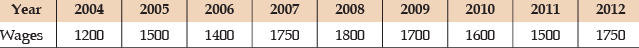Solution: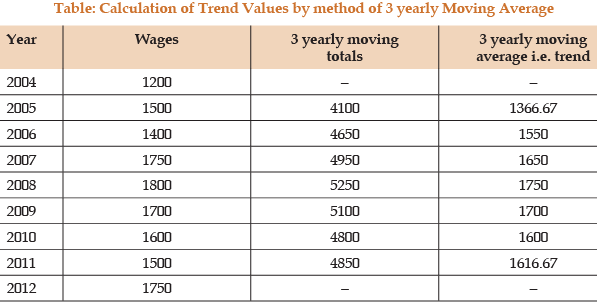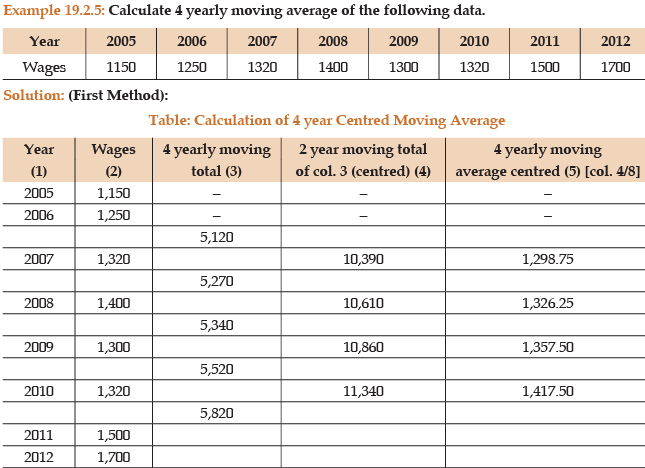Second Method:-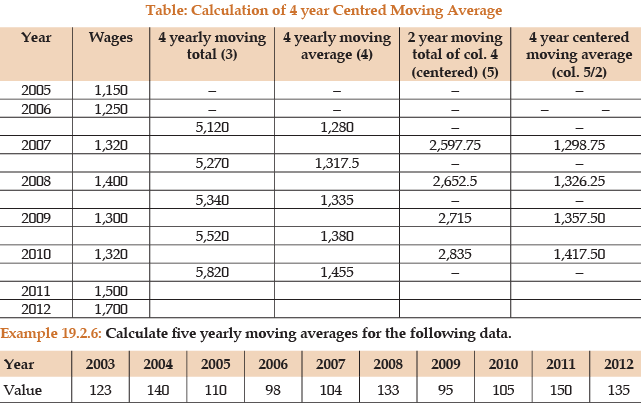Solution:-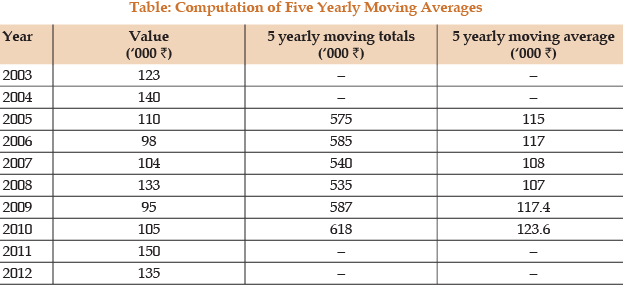The method of least squares as studied in regression analysis can be used to find the trend line of best fit to a time series data.
The regression trend line (Y) is defined by the following equation – Y = a + bX
where Y = predicted value of the dependent variable
a = Y axis intercept or the height of the line above origin (i.e. when X = 0, Y = a)
b = slope of the regression line (it gives the rate of change in Y for a given change in X) (when b is positive the slope is upwards, when b is negative, the slope is downwards) X = independent variable (which is time in this case)
To estimate the constants a and b, the following two equations have to be solved simultaneously –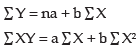To simplify the calculations, if the mid point of the time series is taken as origin, then the negative values in the first half of the series balance out the positive values in the second half so that ∑x = 0. In this case the above two normal equations will be as follows -
∑Y = na
∑XY = b∑X2
In such a case the values of a and b can be calculated as under -
Since ∑Y = na,
Since ∑XY = b∑X2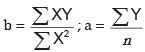Example 19.2.7: Fit a straight line trend to the following data by Least Square Method and estimate the sale for the year 2012.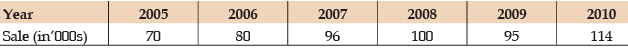Solution: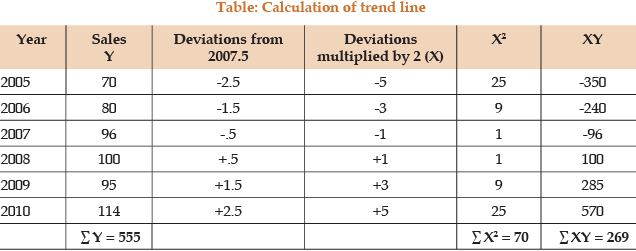N = 6
Equation of the straight line trend is Yo = a + bX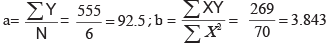Trend equation is Y= 92.5 + 3.843X
For 2012, X = 9
Y2012 = 92.5 + 3.843 × 9 = 92.5 + 34.587
= 126.59 (in ‘000₹)
Example 19.2.8:- Fit a straight line trend to the following data and estimate the likely profit for the year 2012. Also calculate the trend values.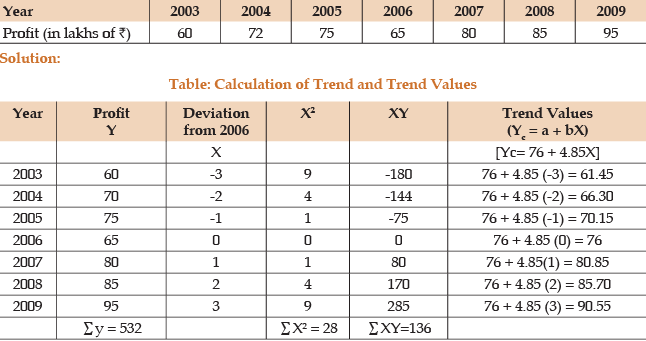N = 7
The equation for straight line trend is Yc = a + bX
Where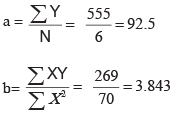The trend equation Yc = 92.5 + 3.843.X
2012, x = 6 (2012 - 2006) Yc = 76 + 4.85(6) = 76 + 29.10
= 105 .10
The estimated profit for the year 2012 is ₹105.10 lakhs.
Example 19.2.9: Calculate Seasonal Indices for each quarter from the following percentages of whole sale price indices to their moving averages.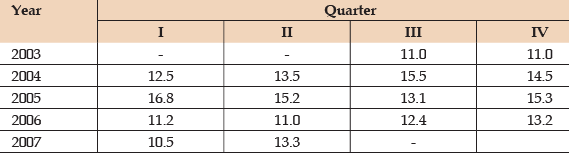Solution:-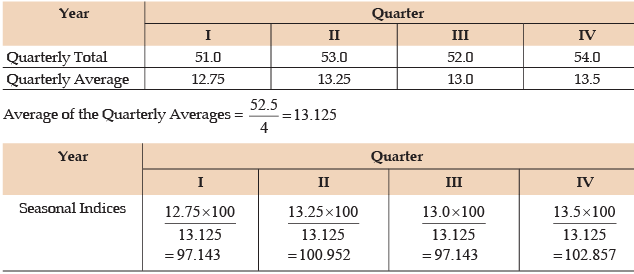Seasonal Indices are calculated by converting the respective quarterly averages on the basis that the average of the quarterly average = 100
Example 19.2.10: Calculate 5- year weighted moving averages for the following data, using weights 1, 1, 3, 2 respectively: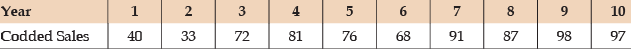Solution :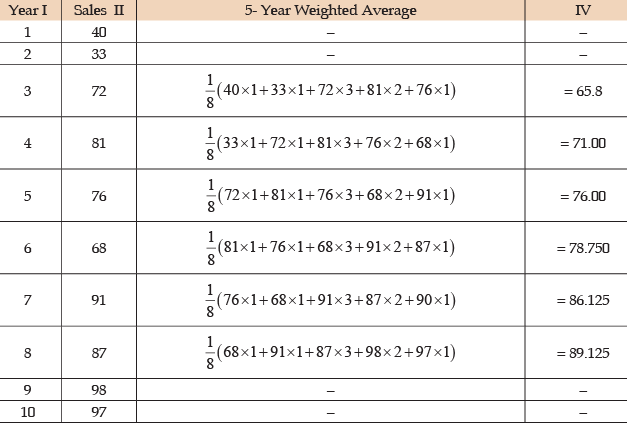Example 19.2.11: Assuming no trend, calculate Seasonal variation indices for the following data.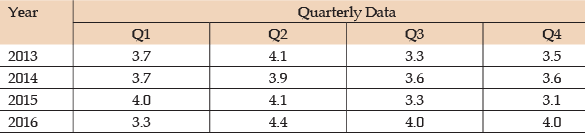Solution: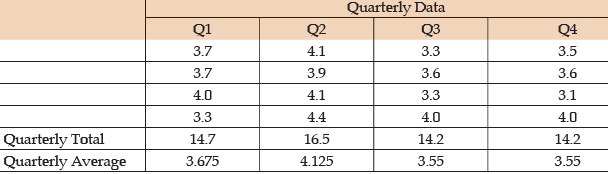Average of Quarterly Averages =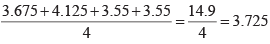Example 19.2.12: Calculate the Seasonal Indices from the following ratio to moving averages values expressed in percentage.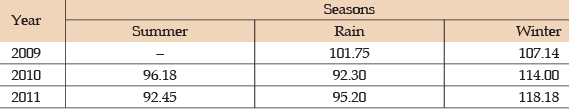Solution: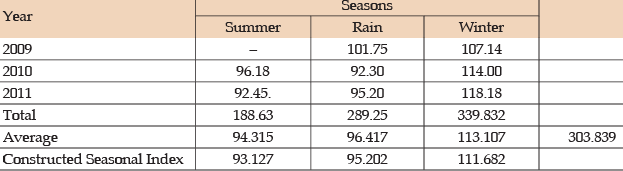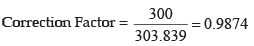Example 19.2.13: From the following data, calculate the trend values, using Four yearly moving average.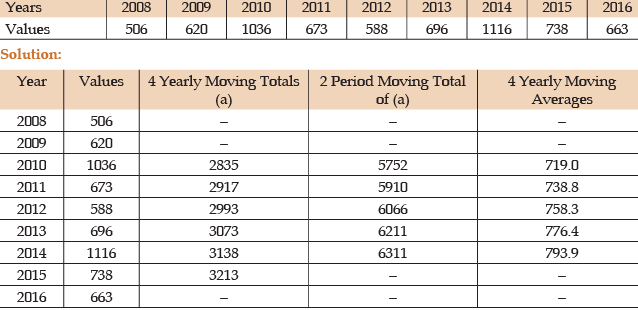Offer running on EduRev: Apply code STAYHOME200 to get INR 200 off on our premium plan EduRev Infinity!

69 docs|76 tests

,

,

,

,

,

,

,

,

,

,

,

,

,

,

,

,

,

,

,

,

,

;